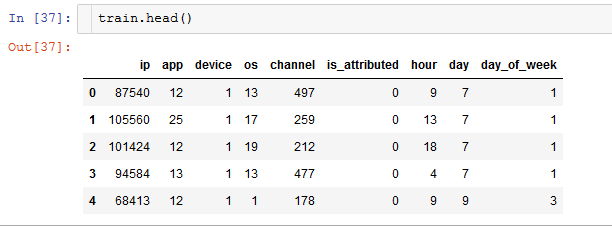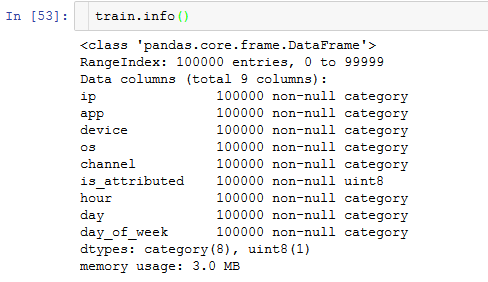# Proc_df problem need at least one array to concatenate [Solved]

I am starting out experimenting with structured data. This is post Lesson 4 and trying to run the proc_df function on my data-frame.

It gives me the following error:

``````---------------------------------------------------------------------------
ValueError                                Traceback (most recent call last)
<ipython-input-52-b85f40a645ed> in <module>()
----> 1 df, y, nas, mapper = proc_df(train, 'is_attributed', do_scale=True)

~/projects/fastai/fastai/structured.py in proc_df(df, y_fld, skip_flds, do_scale, na_dict, preproc_fn, max_n_cat, subset, mapper)
425     if na_dict is None: na_dict = {}
426     for n,c in df.items(): na_dict = fix_missing(df, c, n, na_dict)
--> 427     if do_scale: mapper = scale_vars(df, mapper)
428     for n,c in df.items(): numericalize(df, c, n, max_n_cat)
429     res = [pd.get_dummies(df, dummy_na=True), y, na_dict]

~/projects/fastai/fastai/structured.py in scale_vars(df, mapper)
322         map_f = [([n],StandardScaler()) for n in df.columns if is_numeric_dtype(df[n])]
323         mapper = DataFrameMapper(map_f).fit(df)
--> 324     df[mapper.transformed_names_] = mapper.transform(df)
325     return mapper
326

~/anaconda2/envs/fastai/lib/python3.6/site-packages/sklearn_pandas/dataframe_mapper.py in transform(self, X)
313                 stacked = stacked.toarray()
314         else:
--> 315             stacked = np.hstack(extracted)
316
317         if self.df_out:

~/anaconda2/envs/fastai/lib/python3.6/site-packages/numpy/core/shape_base.py in hstack(tup)
286         return _nx.concatenate(arrs, 0)
287     else:
--> 288         return _nx.concatenate(arrs, 1)
289
290

ValueError: need at least one array to concatenate
``````

I dont really understand what is going on here.
This is while trying to do the Talking Data Kaggle competition which is on right now.

This is how I call it:

`````` df, y, nas, mapper = proc_df(train, 'is_attributed', do_scale=True)
``````

And this is how train looks:Anyone here that can help me understand what is going wrong?
It seems to have to do with the scaling, and I am thinking it could be because there is not enough variance possibly for some of the columns?

1 Like

Ok. This was solved by running:

`train.reset_index(inplace=True)`

Before running proc_df.

2 Likes

Did you ever actually figure out what was causing this issue? I also tried running reset_index but I’m still getting the issue. Also in the Rossmann notebook Jeremy specifically sets the index to ‘date’ just before calling proc_df so it seems like the function should support datetimeIndex.

@saidaspen @sfkiwi
now as the competition ended, how did DNN score for you in this competition please? would be great if you could share the notebook / kernel with the code, to see how you can apply DNN for structured data! i couln’t make the proc_df to work on this data set. had the same issue as above and reset_index wasn’t solving it for me…

thanks…

I’m still facing this issue as well, train.reset_index(inplace=True) didn’t help

Still facing this issue. Can someone (@xtermz, @saidaspen, @miwojc, @sfkiwi ) shed some light on this?

do_scale=False seems to avoid the problem, and it does not seem like the correct solution.

I am working on data that has 5 categorical columns, and a date column (that is set to index as with jeremy’s lesson3-rossman notebook). There are no continuous columns. I am following the lesson3-rossman notebook as a guideline.